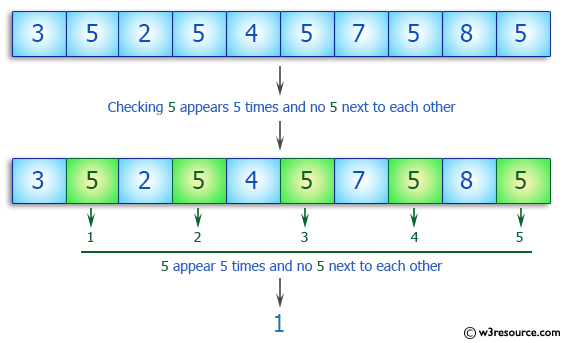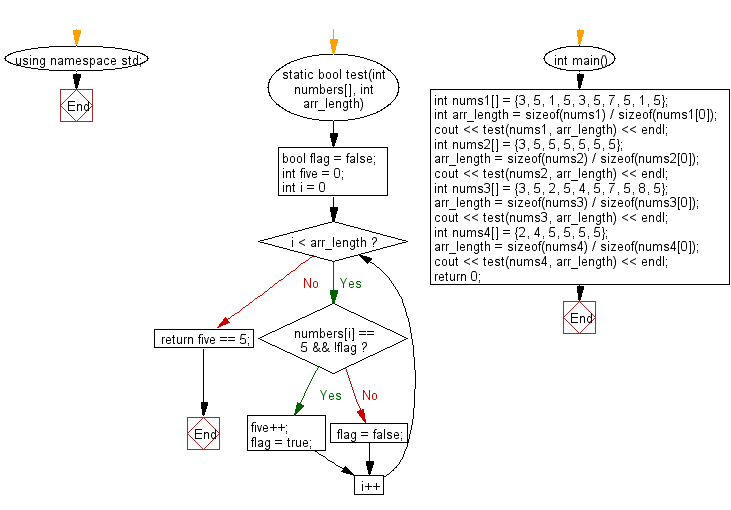﻿ C++ : If 5 appears 5 times, but no 5 next to each other# C++ Exercises: Check a given array of integers and return true if the value 5 appears 5 times and there are no 5 next to each other

## C++ Basic Algorithm: Exercise-112 with Solution

Write a C++ program to check a given array of integers and return true if the value 5 appears 5 times and there are no 5 next to each other.

Sample Solution:

C++ Code :

``````#include <iostream>
using namespace std;

static bool test(int numbers[], int arr_length)
{
bool flag = false;

int five = 0;

for (int i = 0; i < arr_length; i++)
{
if (numbers[i] == 5 && !flag)
{
five++;
flag = true;
}
else
{
flag = false;
}
}

return five == 5;
}

int main()
{
int nums1[] = {3, 5, 1, 5, 3, 5, 7, 5, 1, 5};
int arr_length = sizeof(nums1) / sizeof(nums1);
cout << test(nums1, arr_length) << endl;
int nums2[] = {3, 5, 5, 5, 5, 5, 5};
arr_length = sizeof(nums2) / sizeof(nums2);
cout << test(nums2, arr_length) << endl;
int nums3[] = {3, 5, 2, 5, 4, 5, 7,  5, 8,  5};
arr_length = sizeof(nums3) / sizeof(nums3);
cout << test(nums3, arr_length) << endl;
int nums4[] = {2, 4, 5, 5, 5, 5};
arr_length = sizeof(nums4) / sizeof(nums4);
cout << test(nums4, arr_length) << endl;
return 0;
}
``````

Sample Output:

```1
0
1
0
```

Pictorial Presentation:Flowchart:C++ Code Editor:

Contribute your code and comments through Disqus.

What is the difficulty level of this exercise?

﻿